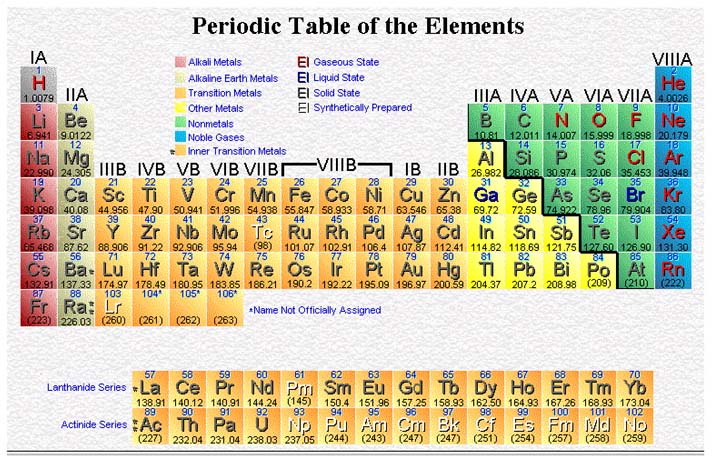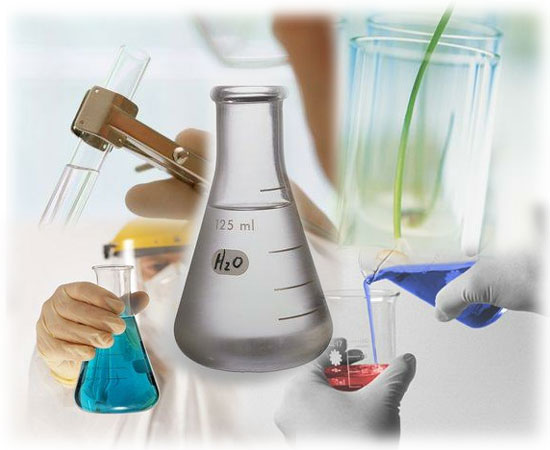CHEMISTRY Pre-AP Unit 17: Oxidation-Reduction ReactionsGoal:

Objectives: The student should be able to:

1. Define and identify the processes of oxidation and reduction.

2. Identify reactions as redox reactions or nonredox reactions.

3. Apply the rules for assigning oxidation numbers in determining the oxidation state of an element within a compound.

4. Identify the substance being oxidized, the substance being reduced, the oxidizing agent, and the reducing agent in a chemical reaction.

5. Predict which elements are strong oxidizing agents and which are strong reducing agents.

6. Use changes in oxidation number to balance redox equations.

7. Recognize the interdependence of oxidation and reduction processes.

8. Derive oxidation and reduction half-reactions from a redox equation.

9. Balance redox equations by the half-reaction method.

Activities:

Homework:

Lecture & Lab Materials (uploaded once per week):

Redox Introduction (lectures for both 4/24 and 4/25)